# AP Calculus AB Practice Test 31

### Test Information10 questions20 minutes

Calculator Disallowed

1.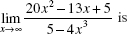2.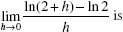3. If y = e-x2, then y (0) equals

4. Use the following table, which shows the values of the differentiable functionsf and g.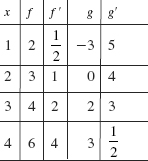The average rate of change of function f on [1,4] is

5. Use the following table, which shows the values of the differentiable functionsf and g.If h(x) = g(f (x)) then h (3) =

6. The derivative of a function f is given for all x by

f (x) = x2(x + 1)3(x - 4)2.

The set of x values for which f is a relative maximum is

7. If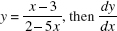equals

8. The maximum value of the function f (x) = xe -x is

9. Which equation has the slope field shown below?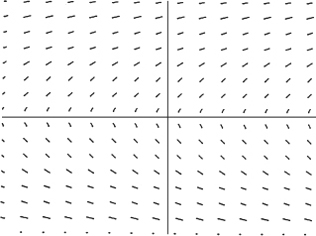10. The graph below shows the velocity of an object moving along a line, for 0 ≤ t ≤ 9.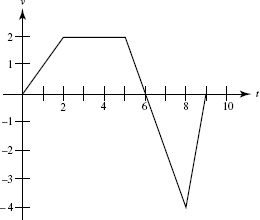At what time does the object attain its maximum acceleration?